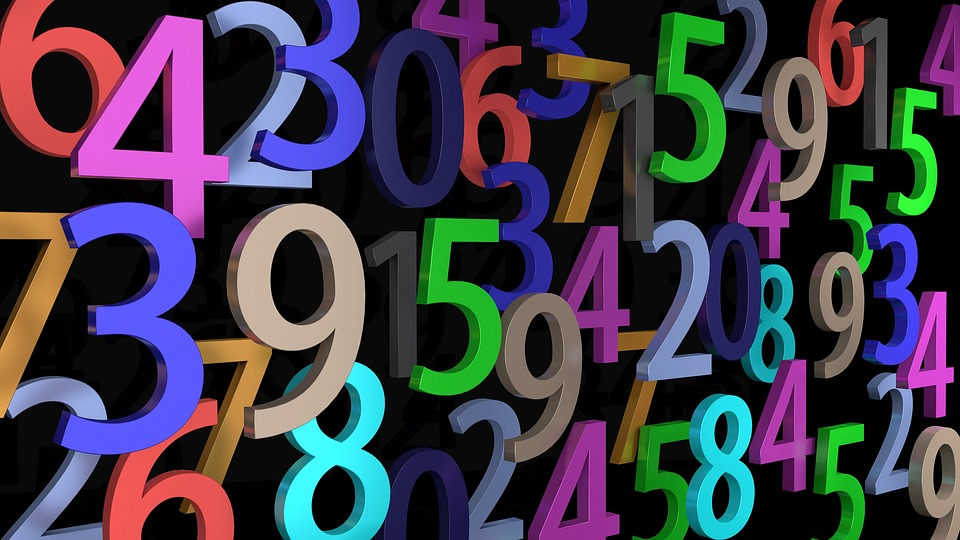# Significant Figures in CalculationsRelated Posts:Source: https://pixabay.com/photos/pay-numbers-digits-mathematics-2662758/

OpenStax Chemistry 2e

The results calculated from a measurement are at least as uncertain as the measurement itself. Take the uncertainty in measurements into account to avoid misrepresenting the uncertainty in calculated results. One way to do this is to report the result of a calculation with the correct number of significant figures, which is determined by the following three rules for rounding numbers:

1. When adding or subtracting numbers, round the result to the same number of decimal places as the number with the least number of decimal places (the least certain value in terms of addition and subtraction).
2. When multiplying or dividing numbers, round the result to the same number of digits as the number with the least number of significant figures (the least certain value in terms of multiplication and division).
3. If the digit to be dropped (the one immediately to the right of the digit to be retained) is less than 5, “round down” and leave the retained digit unchanged; if it is more than 5, “round up” and increase the retained digit by 1. If the dropped digit is 5, and it’s either the last digit in the number or it’s followed only by zeros, round up or down, whichever yields an even value for the retained digit. If any nonzero digits follow the dropped 5, round up. (The last part of this rule may strike you as a bit odd, but it’s based on reliable statistics and is aimed at avoiding any bias when dropping the digit “5,” since it is equally close to both possible values of the retained digit.)

The following examples illustrate the application of this rule in rounding a few different numbers to three significant figures:

• 0.028675 rounds “up” to 0.0287 (the dropped digit, 7, is greater than 5)
• 18.3384 rounds “down” to 18.3 (the dropped digit, 3, is less than 5)
• 6.8752 rounds “up” to 6.88 (the dropped digit is 5, and a nonzero digit follows it)
• 92.85 rounds “down” to 92.8 (the dropped digit is 5, and the retained digit is even)

Let’s work through these rules with a few examples.

##### EXAMPLE 1 Rounding Numbers

Round the following to the indicated number of significant figures:

(a) 31.57 (to two significant figures)

(b) 8.1649 (to three significant figures)

(c) 0.051065 (to four significant figures)

(d) 0.90275 (to four significant figures)

##### Solution

(a) 31.57 rounds “up” to 32 (the dropped digit is 5, and the retained digit is even)

(b) 8.1649 rounds “down” to 8.16 (the dropped digit, 4, is less than 5)

(c) 0.051065 rounds “down” to 0.05106 (the dropped digit is 5, and the retained digit is even)

(d) 0.90275 rounds “up” to 0.9028 (the dropped digit is 5, and the retained digit is even)

##### Check Your Learning

Round the following to the indicated number of significant figures:

(a) 0.424 (to two significant figures)

(b) 0.0038661 (to three significant figures)

(c) 421.25 (to four significant figures)

(d) 28,683.5 (to five significant figures)

##### ANSWER:

(a) 0.42; (b) 0.00387; (c) 421.2; (d) 28,684

EXAMPLE 2 Addition and Subtraction with Significant Figures

Rule: When adding or subtracting numbers, round the result to the same number of decimal places as the number with the fewest decimal places (i.e., the least certain value in terms of addition and subtraction).

(a) Add 1.0023 g and 4.383 g.

(b) Subtract 421.23 g from 486 g.

##### Solution

Answer is 5.385 g (round to the thousandths place; three decimal places)

Answer is 65 g (round to the ones place; no decimal places)

##### Check Your Learning

(a) Add 2.334 mL and 0.31 mL.

(b) Subtract 55.8752 m from 56.533 m.

##### ANSWER:

(a) 2.64 mL; (b) 0.658 m

Source:

Source:

Flowers, P., Theopold, K., Langley, R., & Robinson, W. R. (2019, February 14). Chemistry 2e. Houston, Texas: OpenStax. Access for free at: https://openstax.org/books/chemistry-2e